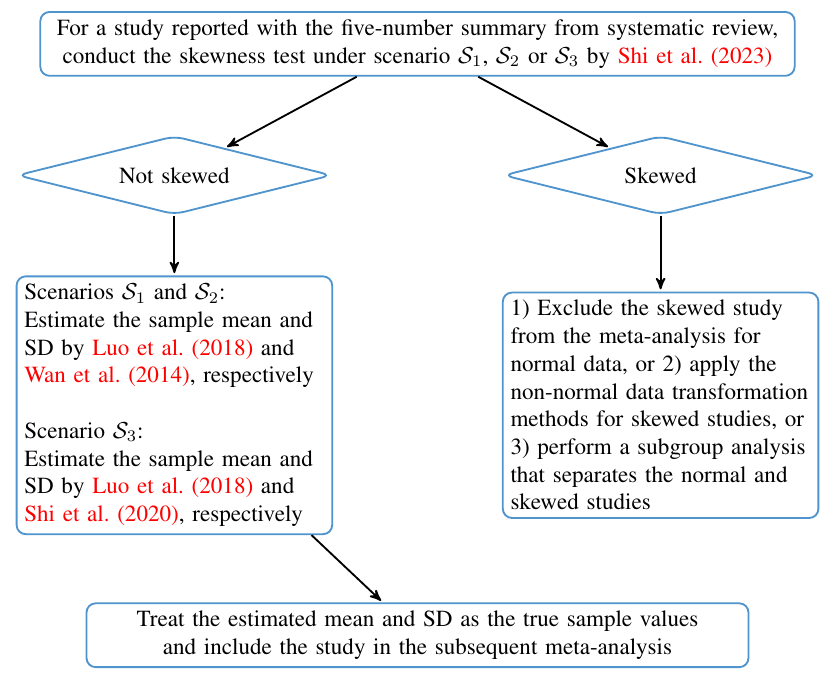## Scenario 1

Size of the sample

Minimum of the sample

Median of the sample

Maximum of the sample

Estimated mean of the sample from Luo et al. (2018)

Estimated standard deviation of the sample from Wan et al. (2014)

## Scenario 2

Size of the sample

First quartile of the sample

Median of the sample

Third quartile of the sample

Estimated mean of the sample from Luo et al. (2018)

Estimated standard deviation of the sample from Wan et al. (2014)

## Scenario 3

Size of the sample

Minimum of the sample

First quartile of the sample

Median of the sample

Third quartile of the sample

Maximum of the sample

Estimated mean of the sample from Luo et al. (2018)

Estimated standard deviation of the sample from Shi et al. (2020)

## References:

J. Shi, D. Luo, X. Wan, Y. Liu, J. Liu, Z. Bian and T. Tong* (2020+), "Detecting the skewness of data from the sample size and the five-number summary", arXiv preprint arXiv:2010.05749.

J. Shi, D. Luo, H. Weng, X. Zeng, L. Lin, H. Chu and T. Tong* (2020), "Optimally estimating the sample standard deviation from the five-number summary", Research Synthesis Methods, 11: 641-654.

D. Luo, X. Wan, J. Liu and T. Tong* (2018), "Optimally estimating the sample mean from the sample size, median, mid-range and/or mid-quartile range", Statistical Methods in Medical Research, 27: 1785-1805.

X. Wan, W. Wang, J. Liu and T. Tong* (2014), "Estimating the sample mean and standard deviation from the sample size, median, range and/or interquartile range", BMC Medical Research Methodology, 14: 135.

## A flow chart for performing meta-analysis when some studies are reported with the whole or part of the five-number summary